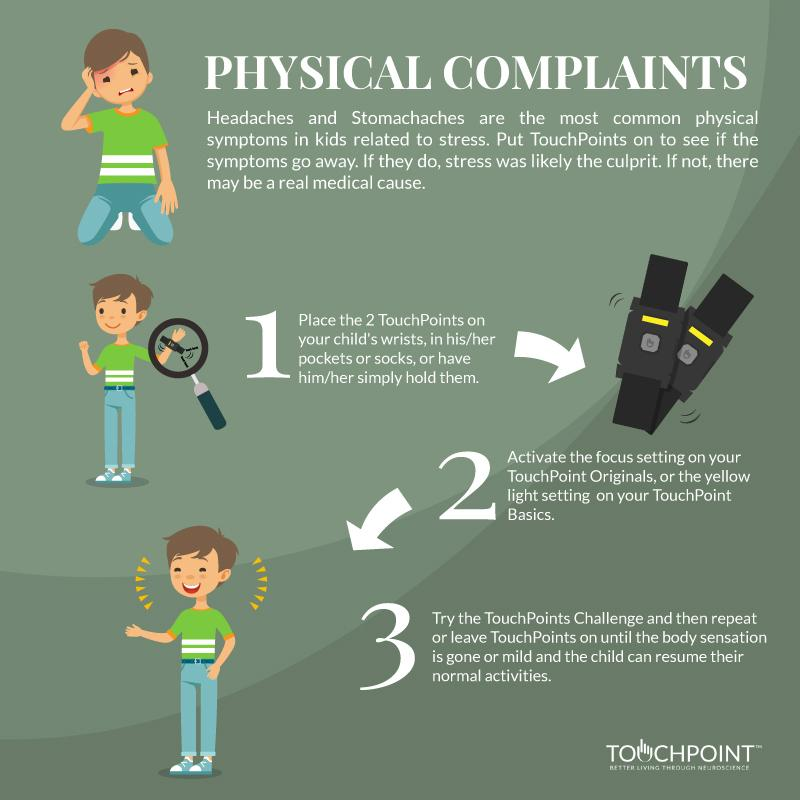0

Your Cart is Empty# Physical Complaints

Headaches and stomachaches are the most common physical symptoms related to stress. Put TouchPoints on to see if the symptoms go away. If they do, stress was likely the culprit. If not, there may be a real medical cause.

### When to use:

Do the TouchPoint Challenge and then repeat or leave TouchPoints on until the body sensation is gone or has become better and you can remove normal activities.

### Which setting to use:

 TouchPoints Original TouchPoints Basic Focus Yellow

### Where to wear TouchPoints:

 Wear on wrists Hold in hands Put in pockets Slide in socks x x x x

<", c, ' onload="var d=', n, ";d.getElementsByTagName('head').", d, "(d.", g, "('script')).", i, "='", a.l, "'\">"].join("") } var c = "body", e = h[c]; if (!e) return setTimeout(q, 100); a.P(1); var d = "appendChild", g = "createElement", i = "src", k = h[g]("div"), l = k[d](h[g]("div")), f = h[g]("iframe"), n = "document", p; k.style.display = "none"; e.insertBefore(k, e.firstChild).id = o + "-" + j; f.frameBorder = "0"; f.id = o + "-frame-" + j; /MSIE[ ]+6/.test(navigator.userAgent) && (f[i] = "javascript:false"); f.allowTransparency = "true"; l[d](f); try { f.contentWindow[n].open() } catch (s) { a.domain = h.domain, p = "javascript:var d=" + n + ".open();d.domain='" + h.domain + "';", f[i] = p + "void(0);" } try { var r = f.contentWindow[n]; r.write(b()); r.close() } catch (t) { f[i] = p + 'd.write("' + b().replace(/"/g, String.fromCharCode(92) + '"') + '");d.close();' } a.P(2) }; a.l && q() })() }(); c[b].lv = "1"; return c[b] } var o = "lightboxjs", k = window[o] = g(o); k.require = g; k.modules = c }({}); /*]]>*/